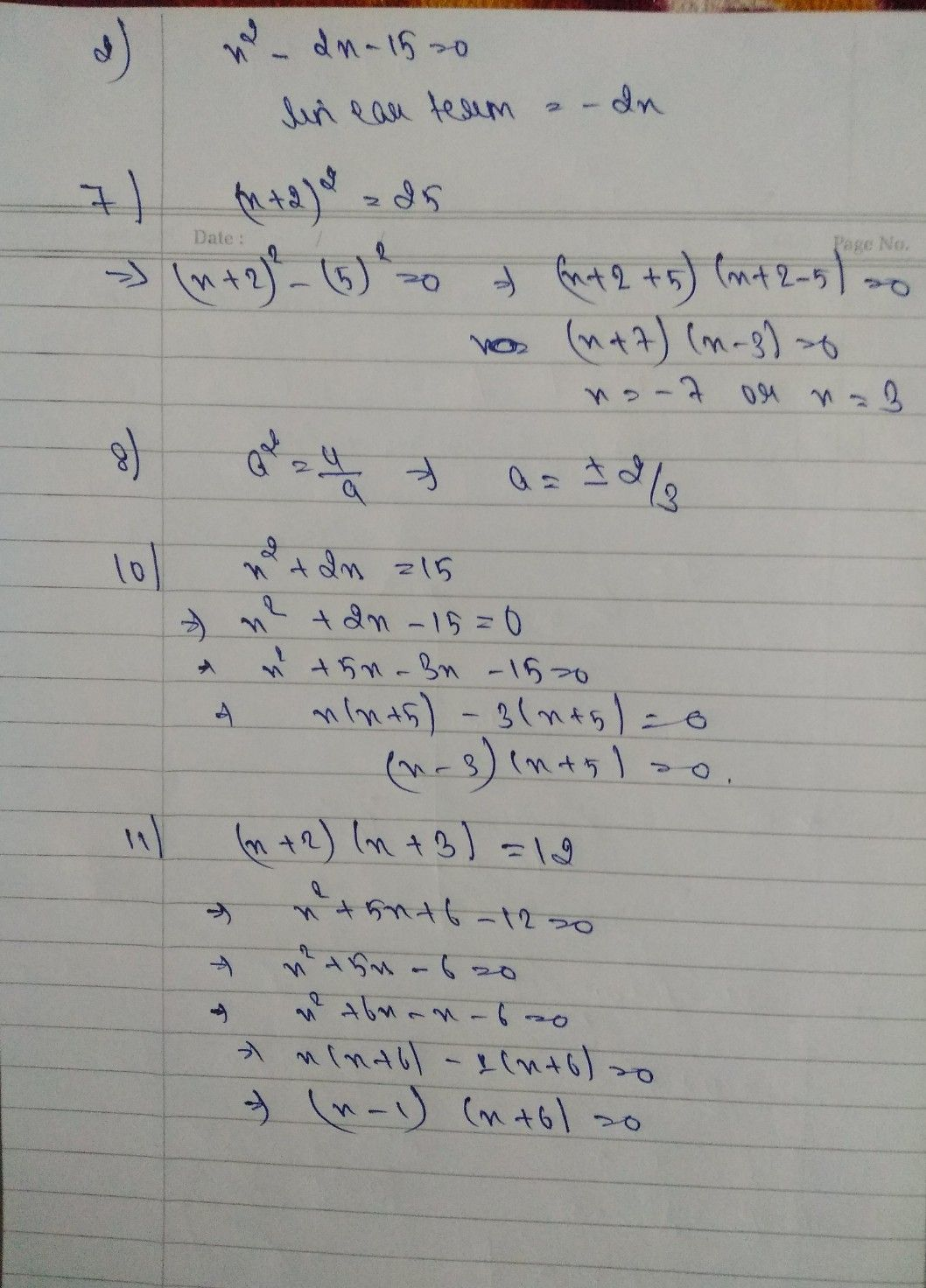Symbol
ProblemNorte 1 kasalu ROQUE NA Camarines 9 SAN Mercedes, MATHEMATICS AS QUIZ SY: $2020-2021$ akatawa ng anc NAME: YEAR & $sECT1ON$ SCORE: PARENT $s1GNAT∪RE:$ wang t. I Direction: Encircle the letter that corresponds to the best answer. 1. It is a polynomial equations of degree two that can be written in the form ax² + bx + c 0 where a, b,c amah are real numbers anda 0. A. Quadratic Inequality C. Quadratic Function ving B. Quadratic Equation D. Linear Equation inup 2. Which is the linear term in the equation of x² - 2x - 15 = 0? hind A.x B. 2x C.-15 D.-2x at u 3. Which of the following is a quadratic equation? A. 2x(x – 3) = 15 C. x3 + 5x + 10 = 0 B. 3m + 8 = 15 D. (n – 2)(m – 2) = 0 4. The following are methods in solving quadratic equations, which one can be used to solve x = 6 + 3? ct A. Extracting square roots C. Completing the square B. Factoring D. Quadratic Formula 5. Which is the solution set given by the equation b2 – 16 = A.-2 and 8 C. 4 and - B. 1 and – 16 D.-8 and 0k? , 6. How many real solutions do we have in the equation x2 %3D if k r= oo0t? . A. Has two real solution or roots. C. Has no real solution or B. Has one real solution or root. D. Cannot be determined 7. Solve: (x + 2)² = 25 A. 5 and 2 B. 6 and 3 4 $C.-7and3$ $2$ D. $-6and4$ 8. What is the solution of the equation a 9. A. ± B. I3 4 $c.±\sqrt{\dfrac {2} {9}}$ $2$ $\sqrt{9}$ $productoftw0$ numbers $iszer0,th$ 9. What property of equality refers to the statement " If the then either of the two is equal to zero or both numbers are equal to zero"? A. Addition property of equality $c.ZeroProduc$ $D.Zer0andNe$ $Pr0perty$ B. Subtraction property of equality Negative $Exp0nen$ 10. Which of the following are factors of the polynomial x2 + $2x=157$ $A.\left(x-3\right)\left(x+5\right)$ $B.\left(x-1\right)\left(x-8\right)$ CD. . $\left(x+1\right)\left(x+8\right)$ $\left(x+3\right)\left(x-5\right)$ 1. What values of xwould make the equation $\left(x+2\right)\left(x+3\right)=12$ $true7$ to mu $A.-2and-3$ C.-1 and 6 3 D.-6 and 1 B. 2 and Some quadratic equations can be solved easily by factoring. To solve such quadratic equations, the Following are procedures except A. Transform the quadratic equation into standard form if necessary. B. Divide the numerical coefficient of the middle term by two then square the quotient. C. Factor the quadratic equation. D. Apply the zero product property by setting each factor of the quadratic expression equal to zera find the solutions of $x^{2}+9x=-8$ by factoring, which of the following procedures is first to be plied? A. Check the values of the variable obtained by substituting each to the original equation. B. Solve each resulting equation. C. Transform the equation to standard $formax^{2}+bx+c=0$ D. Equate both the factors to zero.